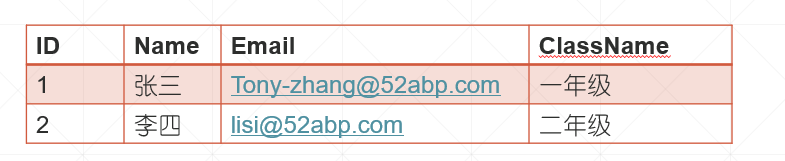## ASP.NET Core MVC 中的 Model 模型_.net core模型都写在哪里-程序员宅基地

### ASP.NET Core MVC 中的 ModelMVC 中的模型包含一组表示数据的类和管理该数据的逻辑。 因此，为了表示我们想要显示的学生数据，我们使用以下 Student 类。

``````public class Student
{
public int Id { get; set; }
public string Name { get; set; }
public string ClassName { get; set; }
}
``````

C#

ASP.NET Core 中的模型类不必位于 Models 文件夹中，但将它们保存在名为 Models 的文件夹中是一种很好的做法，因为以后更容易找到它们。

`````` public interface IStudentRepository
{
Student GetStudent(int id);

}

``````

C#

`````` public class MockStudentRepository : IStudentRepository
{
private List<Student> _studentList;

public MockStudentRepository()
{
_studentList = new List<Student>()
{
new Student() { Id = 1, Name = "张三", ClassName = "一年级", Email = "[email protected]" },
new Student() { Id = 2, Name = "李四", ClassName = "二年级", Email = "[email protected]" },
new Student() { Id = 3, Name = "王二麻子", ClassName = "二年级", Email = "[email protected]" },
};
}

public Student GetStudent(int id)
{
return _studentList.FirstOrDefault(a => a.Id == id);
}
}
``````

C#